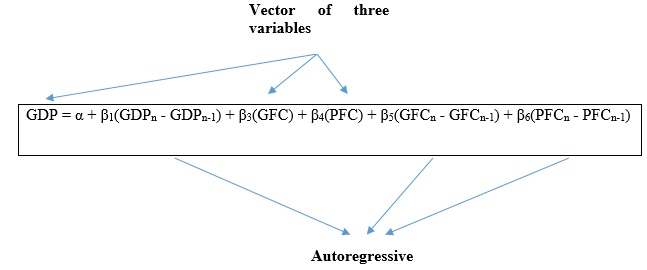# How to perform regression analysis using VAR in STATA?

The previous article on time series analysis showed how to perform Autoregressive Integrated Moving Average (ARIMA) on the Gross Domestic Product (GDP) of India for the period 1996 – 2016 using STATA. The underlining feature of ARIMA is that it studies the behaviour of univariate time series like GDP over a specified time period. Based on that, it recommends an ARIMA equation. This equation then helps to forecast the Gross Domestic Product (GDP) for further years. However, ARIMA is insufficient in defining an econometrics model with more than one variable.  For instance, to find the effect of Gross Fixed Capital Formation (GFC) and Private Final Consumption (PFC) on the GDP, ARIMA is not the correct approach. That is where multivariate time series is useful. Consequently, this article explains the process of performing a regression analysis using vector Auto-Regression (VAR) in STATA.

## Equation of Vector Auto-Regression (VAR)

In multivariate time series, the prominent method of regression analysis is Vector Auto-Regression (VAR). It is important to understand VAR for more clarity. Firstly, the term ‘auto-regression’ is used due to the appearance of the lagged value of dependent variables on the right side. Secondly, the term ‘vector’ refers to dealing with the vector of two or more variables. The resultant equation will be as follows:Figure 1: Equation of VAR

In the above VAR equation, all three variables are inter-related and simultaneously achieved. Since GFC and PFC play a role in the calculation of GDP, the simultaneity between these variables are universal.

To proceed with VAR in STATA, it is important to recognize all the steps, assumptions and important tests in the process.

## Steps in performing VAR in STATA

 1. Lag selection of Variables As noted in the above equation, the variables are interrelated with lagged values of other variables. However, it is unclear how many lags the variables show interrelation. Therefore, to begin VAR, first, it is imperative to recognize the exact level of lags at which variables are inter-connected or endogenously obtained. 2.      Stationarity In the previous articles the time series data showed that GDP is non-stationary. Therefore it uses the first differencing. The same case could also happen for GFC and PFC. Therefore, the second step would be to check and assure stationarity in data. 3. Test for Co-integration In case of co-integration, suppose there are two or more non-stationary variables for regression. While estimating residuals from the regression, the residuals turn out to be stationary. That means, two or more non-stationary series may result in a stationary series. This is called as co-integration. The implication of co-integration is that two variables have a long-term casualty and in long run, the variables might converge towards an equilibrium value. Equilibrium value is steady, therefore they have equal means and variance, or ‘stationary’. Therefore, before initiating VAR, find out if the present model contains any co-integration or equilibrium state. Co-integration indicates a long-term association between two or more non-stationary variables. 4. If Co-integration is not present = We apply VAR. VAR technique where variables are endogenous and dependent on lagged values of other variables. 5. If co-integration is present = apply Vector Error Correction Model (VECM). VECM model takes into account the long term and short term causality dynamics. It also offers a possibility to apply VAR to integrated multivariate time series. 6. VECM diagnostic, tests and forecasting Based on the constructed VECM model, review the assumptions of autocorrelation and normality, and then proceed to forecast. 7. ARCH (Autoregressive Conditionally Heteroscedastic Model) Time series models incorporating the effects of volatility. 8. Extensions of ARCH GARCH (Generalized Autoregressive Conditional Heteroskedasticity) and T-GARCH (Threshold- Generalized Autoregressive Conditional Heteroskedasticity).

Table 1: Tests of VAR Models

The next article shows lag selection in a VAR model involving two variables GDP and PFC.

### Priya Chetty

Partner at Project Guru
Priya is a master in business administration with majors in marketing and finance. She is fluent with data modelling, time series analysis, various regression models, forecasting and interpretation of the data. She has assisted data scientists, corporates, scholars in the field of finance, banking, economics and marketing.

#### Latest posts by Priya Chetty (see all)

Discussions

1.Belay Zewude
2.Sahar